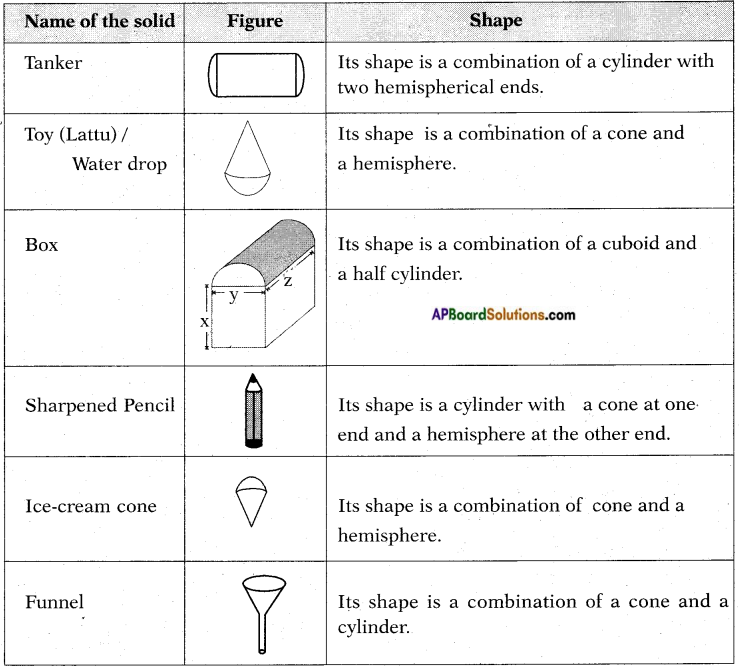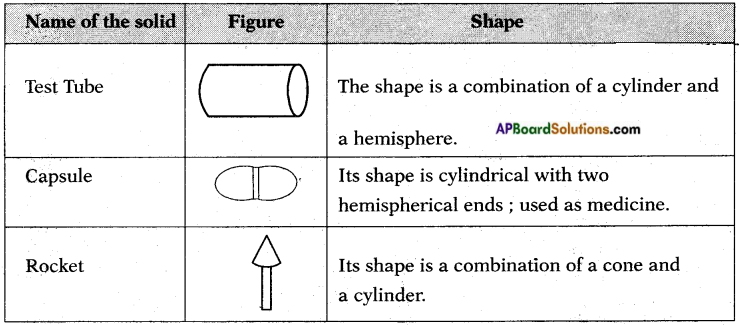Students can go through AP SSC 10th Class Maths Notes Chapter 10 Mensuration to understand and remember the concepts easily.

## AP State Syllabus SSC 10th Class Maths Notes Chapter 10 Mensuration

→ A solid is a geometrical shape with three dimensions namely length, breadth and height.
Eg: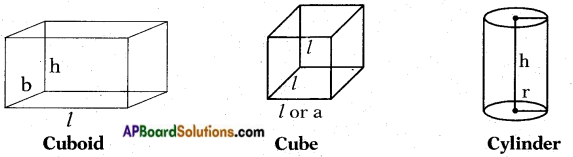→ A solid has two types of area namely,
a) Lateral Surface Area (L.S.A.)
b) Total Surface Area (T.S.A,)

→ In general, L.S.A. of a solid is the product of its base perimeter and height.
Eg : L.S.A. of a cuboid = 2h(l + b)
L.S.A. of a cylinder = 2πrh

→ The T.S.A. of a solid is the sum of L.S.A. and the areas of its top and base.
Eg : T.S.A. of a cylinder = 2πrh + 2πr2
= 2πr(r + h)

→ In general, the volume of a solid is the product of its base area and height.
V = A. h
Eg: Volume of a cube = a2 . a = a3
Volume of a cylinder = πr2 . h = πr2h→ The volume of solid formed by joining two basic solids is the sum of volumes of the constituents.

→ Surface area of the combination of solids: In calculating the surface area of the solid which is a combination of two or more solids, we can’t add the surface areas of all its constituents, because some part of the surface area disappears in the process of joining them.

→ Surface areas and volume of different solid shapes: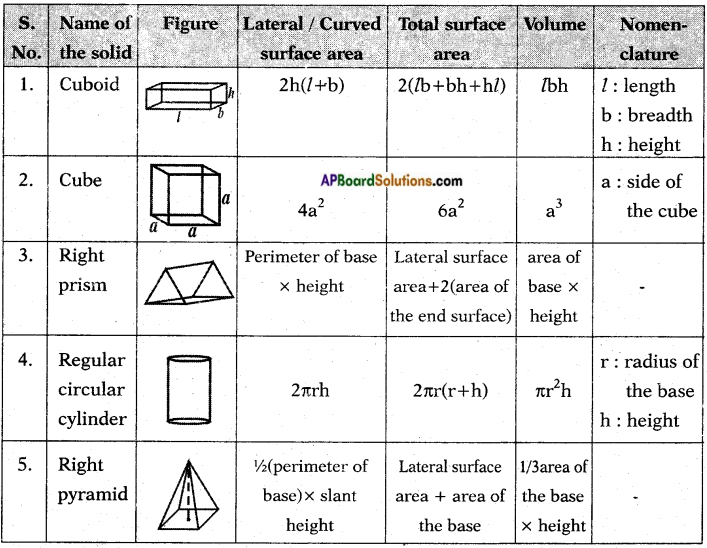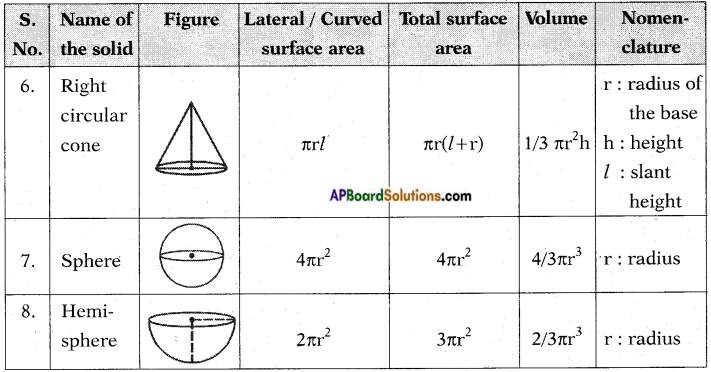→ Some solid figures and their combination shapes: